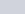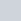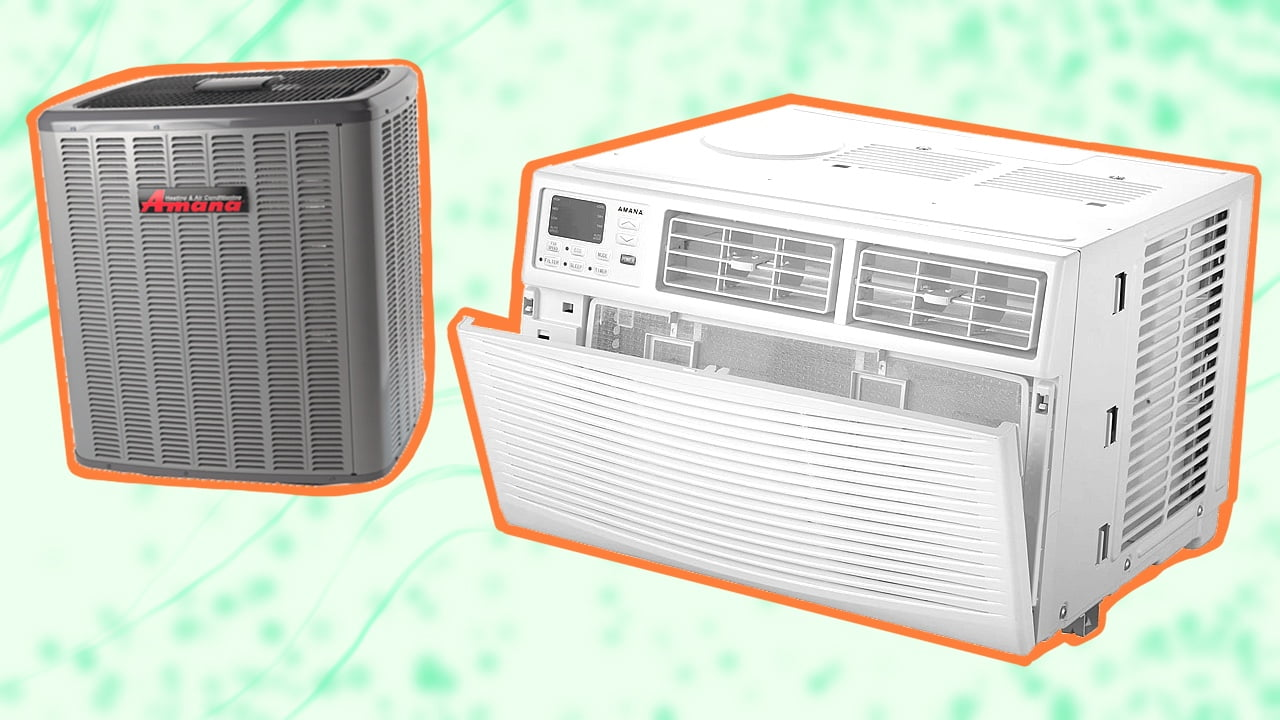X# Watts To Amps Converter: Guide To Your House Electrical NetworkWatts is a measure of the amount of energy being released. It is created when amps and volts combine. The higher the wattage, the more will be the output and power from an appliance. A watts-to-amps calculator converts watts (electric power) to amps (electric current) in alternating current (AC) and direct current (DC) power systems.

## Watts to Amps Calculator

Amps to Watts

Switch

Results:

Watts to Amps

Switch

Results:

You can easily convert watts to amps (amperage) by using the electrical power formula, according to which power (watts) is divided by voltage (volts) to get amps. The formula for this is:

Amps (I)=Watts (P) Voltage (V)

This formula is applicable only for devices that operate with DC power, whereas for AC current, the power factor is considered. So, the equation goes:

Amps (I)=Watts (P)Voltage (V) Power Factor (PF)

## Watts To Amps Converter: Some Key Terms ExplainedBefore you can fully understand why the conversion from watts to amps is important, you must have a brief idea of certain terms associated with this formula. Let me tell you more about them:

### Volts

Volt or voltage is the measure of force or electric potential. Simply put, it helps measure how much force is required to get the electrical current flowing. In the United States, home appliances work at different voltages. For instance, air conditioning units, clothes dryers, etc., operate at 240 volts, whereas computers, light bulbs, and phone chargers work at an electrical supply of 120 volts.

Before making a big investment, like buying a new air conditioner, you must check your AC system’s cooling capacity. You can do so using BTU to kW air conditioning calculator or even the kW to BTU calculator.

### Amps

Amps or ampere is a unit that measures electrical current. It determines the amount of electricity that runs through a circuit. In a circuit breaker, you will often notice the amperage written, and the larger this number, the more electricity can flow through the circuit.

To calculate amps, you divide watts by volts. So, if you have an 800W electric appliance connected to a circuit of 240V, then it will be drawing 3.33 A.

Similarly, you can calculate how many amps of current are drawn per watt of power using the amps formula. For example, at 120 volts, you will need 8.33 amps of current to generate 1000 watts of power.

### Watts

I’ve already explained what watts are. To recap, it represents the energy produced when volts and amps work together. It is the power that an electric device uses to run. For instance, when you light a bulb or run a vacuum, it consumes a certain power, which is expressed in watts.

You can calculate watts by multiplying amps with volts. For example, at 120 volts, 1 amp will produce 120 watts, and at 120 volts, 20 amps will produce 2,400 watts.

In larger units, the power consumed is mentioned in kilowatts. One kW = 1000 watts.

## Convert Watts To Amps: All You Need To Know

When you are converting watts to amps, the first step is to know what is the current type you are dealing with.

In DC or direct current, the electric current flows in one direction. This type of current is produced by solar panels and used by devices (such as laptops, cell phones, etc.) that run on the electric battery. When the battery is connected to a circuit, electric charge flows in a single direction from negative charge to positive charge.

AC or alternating current has current flowing in both directions. Usually, the current changes direction periodically. The AC supply is used extensively in homes and businesses.

Tip

Although the calculation is easy to do manually, I recommend using an online calculator instead. When you use the watts to amps calculator, you will be asked to insert a few values.

For instance, you will be asked to specify the current type you’re working with – DC, AC (single), or AC (three-phase). Then, you will be asked to insert the amps-watts-volts values. For an AC voltage, you will be given the power factor (usually taken as 1).

### Watts To Amps Conversion For AC Single Phase

For DC watts to amps calculation, you have to divide power (expressed in watts) by the power factor times RMS voltage (expressed in volts). So:

Amps (I)=Watts (P)Voltage (V) Power Factor (PF)

## Watts To Amps Conversion For AC Three Phase

#### For three-phase units, you will get the option to choose between the line-to-line voltage and line-to-neutral voltage. The PF is taken as one, and a certain square root is derived.

You simply have to insert the given values and choose the voltage type, and the results will be calculated for you.## Final Words

Converting watts to amps may feel simple, but there are a lot of factors you need to consider. It is not simply possible to convert watts directly to amperes or amps to watts. Instead, you must know the resistance (r), voltage, voltage type, and so on. This is why it is best to use a calculator.

Calculators designed for electrical systems, such as BTU calculators, also come in handy when trying to determine a home appliance’s cooling capacity or when trying to convert between EER and COP numbers.

With that, I will take my leave. Goodbye!